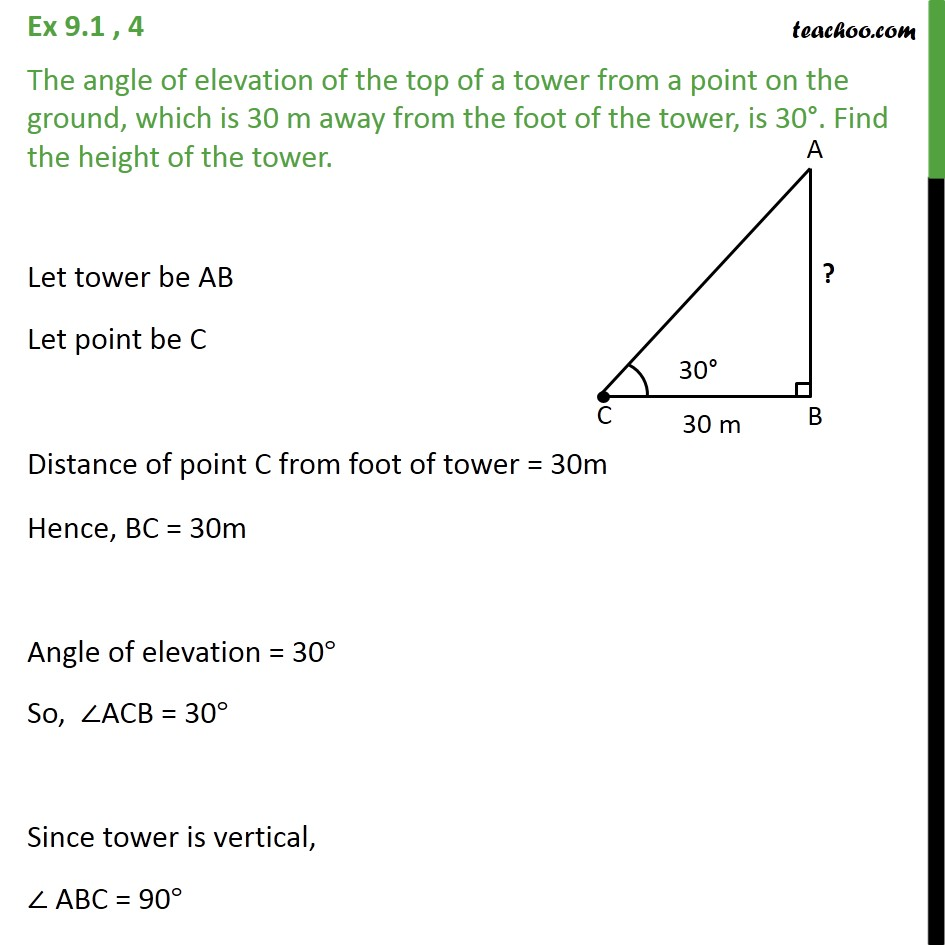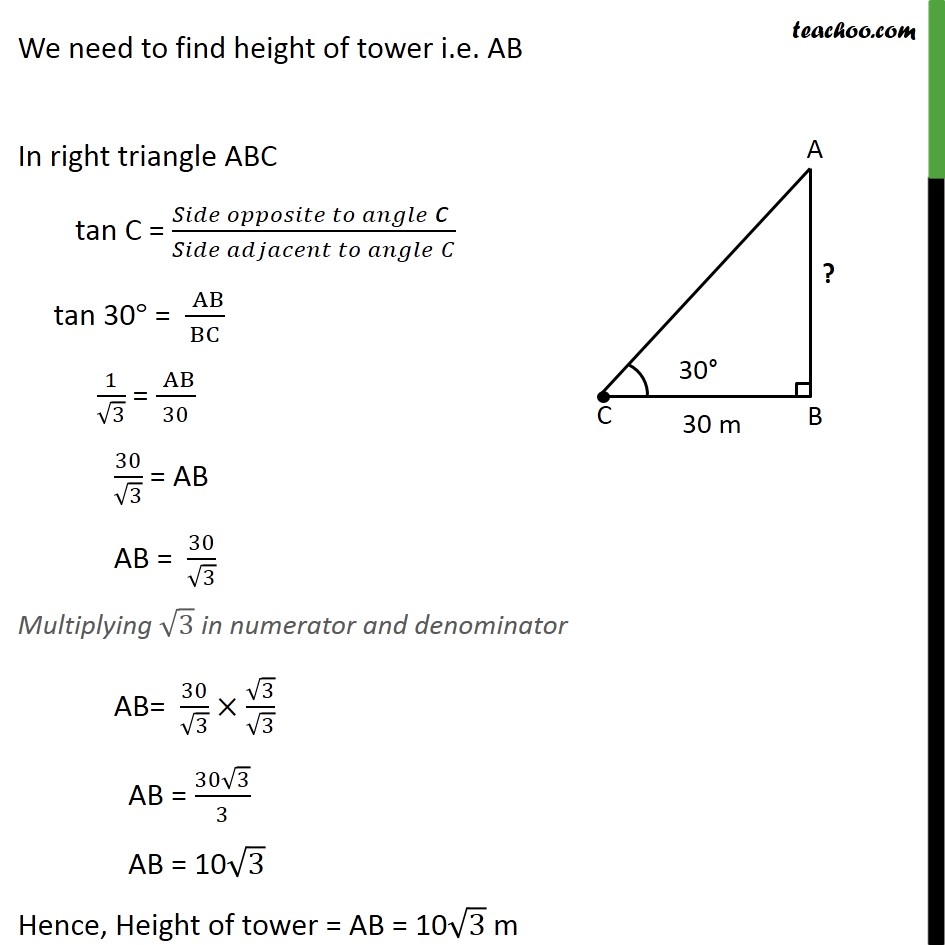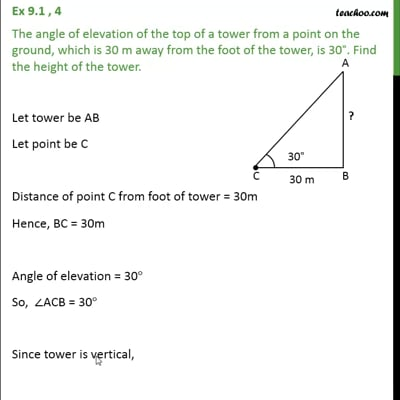Ex 9.1

Chapter 9 Class 10 Some Applications of Trigonometry
Serial order wiseThis video is only available for Teachoo black users

Maths Crash Course - Live lectures + all videos + Real time Doubt solving!

### Transcript

Ex 9.1 , 4 The angle of elevation of the top of a tower from a point on the ground, which is 30 m away from the foot of the tower, is 30°. Find the height of the tower. Let tower be AB Let point be C Distance of point C from foot of tower = 30m Hence, BC = 30m Angle of elevation = 30° So, ∠ACB = 30° Since tower is vertical, ∠ ABC = 90° We need to find height of tower i.e. AB In right triangle ABC tan C = (𝑆𝑖𝑑𝑒 𝑜𝑝𝑝𝑜𝑠𝑖𝑡𝑒 𝑡𝑜 𝑎𝑛𝑔𝑙𝑒" c " )/(𝑆𝑖𝑑𝑒 𝑎𝑑𝑗𝑎𝑐𝑒𝑛𝑡 𝑡𝑜 𝑎𝑛𝑔𝑙𝑒" " 𝐶) tan 30° = (" " AB)/BC 1/√3 = (" " AB)/30 30/√3 = AB AB = 30/√3 Multiplying √3 in numerator and denominator AB= 30/√3 × √3/√3 AB = (30√3)/3 AB = 10√3 Hence, Height of tower = AB = 10√3 m